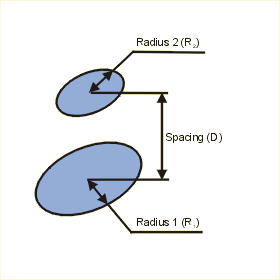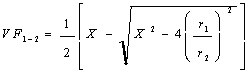Close

This page calculates the net radiant heat exchange for two isothermal (constant temperature) parallel flat black disks.The heat flow (q) from the plate is calculated as:

q = 56.69x10-9 x VF(1-2) x A1 x (T14 - T24)

Where 56.69x10-9 is the average Stefan-Bolzmann constant, A1 is the area of disk 1, T1 is the disk 1 temperature and T2 is the disk 2 temperature. The view factor VF(1-2) (also known as radiation shape factor, angle factor, and configuration factor) is defined as:Where r1, r2 and X are defined as: